9 Linear Momentum and Collisions

# 9.7 Rocket Propulsion

### Learning Objectives

By the end of this section, you will be able to:

• Describe the application of conservation of momentum when the mass changes with time, as well as the velocity
• Calculate the speed of a rocket in empty space, at some time, given initial conditions
• Calculate the speed of a rocket in Earth’s gravity field, at some time, given initial conditions

Now we deal with the case where the mass of an object is changing. We analyze the motion of a rocket, which changes its velocity (and hence its momentum) by ejecting burned fuel gases, thus causing it to accelerate in the opposite direction of the velocity of the ejected fuel (see Figure). Specifically: A fully fueled rocket ship in deep space has a total mass ${m}_{0}$ (this mass includes the initial mass of the fuel). At some moment in time, the rocket has a velocity $\mathbf{\overset{\to }{v}}$ and mass m; this mass is a combination of the mass of the empty rocket and the mass of the remaining unburned fuel it contains. (We refer to m as the “instantaneous mass” and $\mathbf{\overset{\to }{v}}$ as the “instantaneous velocity.”) The rocket accelerates by burning the fuel it carries and ejecting the burned exhaust gases. If the burn rate of the fuel is constant, and the velocity at which the exhaust is ejected is also constant, what is the change of velocity of the rocket as a result of burning all of its fuel?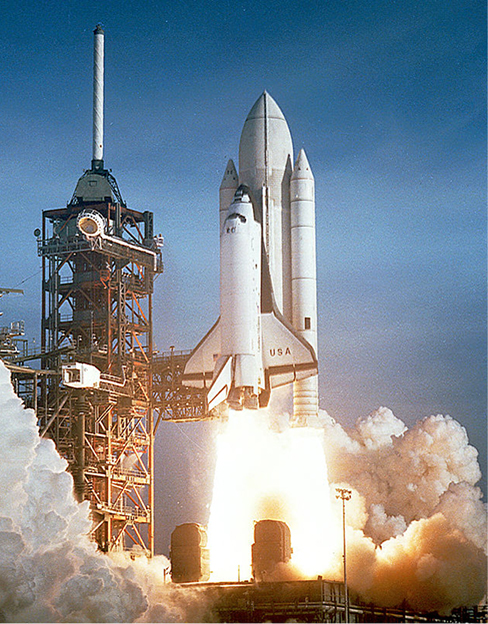Figure 9.32 The space shuttle had a number of reusable parts. Solid fuel boosters on either side were recovered and refueled after each flight, and the entire orbiter returned to Earth for use in subsequent flights. The large liquid fuel tank was expended. The space shuttle was a complex assemblage of technologies, employing both solid and liquid fuel, and pioneering ceramic tiles as reentry heat shields. As a result, it permitted multiple launches as opposed to single-use rockets. (credit: modification of work by NASA)

### Physical Analysis

Here’s a description of what happens, so that you get a feel for the physics involved.

• As the rocket engines operate, they are continuously ejecting burned fuel gases, which have both mass and velocity, and therefore some momentum. By conservation of momentum, the rocket’s momentum changes by this same amount (with the opposite sign). We will assume the burned fuel is being ejected at a constant rate, which means the rate of change of the rocket’s momentum is also constant. By Figure, this represents a constant force on the rocket.
• However, as time goes on, the mass of the rocket (which includes the mass of the remaining fuel) continuously decreases. Thus, even though the force on the rocket is constant, the resulting acceleration is not; it is continuously increasing.
• So, the total change of the rocket’s velocity will depend on the amount of mass of fuel that is burned, and that dependence is not linear.

The problem has the mass and velocity of the rocket changing; also, the total mass of ejected gases is changing. If we define our system to be the rocket + fuel, then this is a closed system (since the rocket is in deep space, there are no external forces acting on this system); as a result, momentum is conserved for this system. Thus, we can apply conservation of momentum to answer the question (Figure).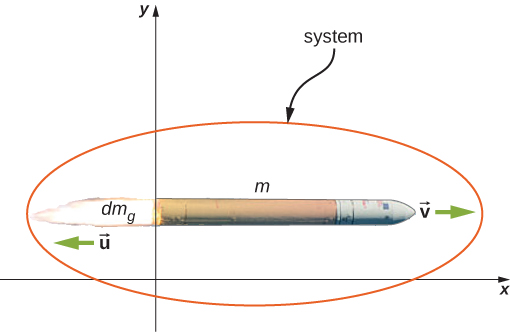Figure 9.33 The rocket accelerates to the right due to the expulsion of some of its fuel mass to the left. Conservation of momentum enables us to determine the resulting change of velocity. The mass m is the instantaneous total mass of the rocket (i.e., mass of rocket body plus mass of fuel at that point in time). (credit: modification of work by NASA/Bill Ingalls)

At the same moment that the total instantaneous rocket mass is m (i.e., m is the mass of the rocket body plus the mass of the fuel at that point in time), we define the rocket’s instantaneous velocity to be $\mathbf{\overset{\to }{v}}=v\mathbf{\hat{i}}$ (in the +x-direction); this velocity is measured relative to an inertial reference system (the Earth, for example). Thus, the initial momentum of the system is

${\mathbf{\overset{\to }{p}}}_{\text{i}}=mv\mathbf{\hat{i}}.$

The rocket’s engines are burning fuel at a constant rate and ejecting the exhaust gases in the −x-direction. During an infinitesimal time interval dt, the engines eject a (positive) infinitesimal mass of gas $d{m}_{g}$ at velocity $\mathbf{\overset{\to }{u}}=\text{−}u\mathbf{\hat{i}}$; note that although the rocket velocity $v\mathbf{\hat{i}}$ is measured with respect to Earth, the exhaust gas velocity is measured with respect to the (moving) rocket. Measured with respect to the Earth, therefore, the exhaust gas has velocity $(v-u)\mathbf{\hat{i}}$.

As a consequence of the ejection of the fuel gas, the rocket’s mass decreases by $d{m}_{g}$, and its velocity increases by $dv\mathbf{\hat{i}}$. Therefore, including both the change for the rocket and the change for the exhaust gas, the final momentum of the system is

$\begin{array}{cc}\hfill {\mathbf{\overset{\to }{p}}}_{\text{f}}& ={\mathbf{\overset{\to }{p}}}_{\text{rocket}}+{\mathbf{\overset{\to }{p}}}_{\text{gas}}\hfill \\ & =(m-d{m}_{g})(v+dv)\mathbf{\hat{i}}+d{m}_{g}(v-u)\mathbf{\hat{i}}\hfill \end{array}\text{.}$

Since all vectors are in the x-direction, we drop the vector notation. Applying conservation of momentum, we obtain

$\begin{array}{l}{p}_{\text{i}}={p}_{\text{f}}\\ mv=(m-d{m}_{g})(v+dv)+d{m}_{g}(v-u)\\ mv=mv+mdv-d{m}_{g}v-d{m}_{g}dv+d{m}_{g}v-d{m}_{g}u\\ mdv=d{m}_{g}dv+d{m}_{g}v.\end{array}$

Now, $d{m}_{g}$ and dv are each very small; thus, their product $d{m}_{g}dv$ is very, very small, much smaller than the other two terms in this expression. We neglect this term, therefore, and obtain:

$mdv=d{m}_{g}u.$

Our next step is to remember that, since $d{m}_{g}$ represents an increase in the mass of ejected gases, it must also represent a decrease of mass of the rocket:

$d{m}_{g}=\text{−}dm.$

Replacing this, we have

$mdv=\text{−}dmu$

or

$dv=\text{−}u\frac{dm}{m}.$

Integrating from the initial mass mi to the final mass m of the rocket gives us the result we are after:

$\begin{array}{ccc}\hfill {\int }_{{v}_{\text{i}}}^{v}dv& =\hfill & \text{−}u{\int }_{{m}_{\text{i}}}^{m}\frac{1}{m}dm\hfill \\ \hfill v-{v}_{\text{i}}& =\hfill & u\,\text{ln}(\frac{{m}_{\text{i}}}{m})\hfill \end{array}$

and thus our final answer is

$\Delta v=u\,\text{ln}(\frac{{m}_{\text{i}}}{m}).$

This result is called the rocket equation. It was originally derived by the Soviet physicist Konstantin Tsiolkovsky in 1897. It gives us the change of velocity that the rocket obtains from burning a mass of fuel that decreases the total rocket mass from ${m}_{0}$ down to m. As expected, the relationship between $\Delta v$ and the change of mass of the rocket is nonlinear.

### Problem-Solving Strategy: Rocket Propulsion

In rocket problems, the most common questions are finding the change of velocity due to burning some amount of fuel for some amount of time; or to determine the acceleration that results from burning fuel.

1. To determine the change of velocity, use the rocket equation Figure.
2. To determine the acceleration, determine the force by using the impulse-momentum theorem, using the rocket equation to determine the change of velocity.

### Example

#### Thrust on a Spacecraft

A spacecraft is moving in gravity-free space along a straight path when its pilot decides to accelerate forward. He turns on the thrusters, and burned fuel is ejected at a constant rate of $2.0\times {10}^{2}\,\text{kg/s}$, at a speed (relative to the rocket) of $2.5\times {10}^{2}\,\text{m/s}$. The initial mass of the spacecraft and its unburned fuel is $2.0\times {10}^{4}\,\text{kg}$, and the thrusters are on for 30 s.

1. What is the thrust (the force applied to the rocket by the ejected fuel) on the spacecraft?
2. What is the spacecraft’s acceleration as a function of time?
3. What are the spacecraft’s accelerations at t = 0, 15, 30, and 35 s?

#### Strategy

1. The force on the spacecraft is equal to the rate of change of the momentum of the fuel.
2. Knowing the force from part (a), we can use Newton’s second law to calculate the consequent acceleration. The key here is that, although the force applied to the spacecraft is constant (the fuel is being ejected at a constant rate), the mass of the spacecraft isn’t; thus, the acceleration caused by the force won’t be constant. We expect to get a function a(t), therefore.
3. We’ll use the function we obtain in part (b), and just substitute the numbers given. Important: We expect that the acceleration will get larger as time goes on, since the mass being accelerated is continuously decreasing (fuel is being ejected from the rocket).

#### Solution

1. The momentum of the ejected fuel gas is
$p={m}_{g}v.$

The ejection velocity $v=2.5\times {10}^{2}\text{m/s}$ is constant, and therefore the force is

$F=\frac{dp}{dt}=v\frac{d{m}_{g}}{dt}=\text{−}v\frac{dm}{dt}.$

Now, $\frac{d{m}_{g}}{dt}$ is the rate of change of the mass of the fuel; the problem states that this is $2.0\times {10}^{2}\text{kg/s}$. Substituting, we get

$\begin{array}{cc}\hfill F& =v\frac{d{m}_{g}}{dt}\hfill \\ & =(2.5\times {10}^{2}\,\frac{\text{m}}{\text{s}})(2.0\times {10}^{2}\,\frac{\text{kg}}{\text{s}})\hfill \\ & =5\times {10}^{4}\,\text{N.}\hfill \end{array}$
2. Above, we defined m to be the combined mass of the empty rocket plus however much unburned fuel it contained: $m={m}_{R}+{m}_{g}$. From Newton’s second law,
$a=\frac{F}{m}=\frac{F}{{m}_{R}+{m}_{g}}.$

The force is constant and the empty rocket mass ${m}_{R}$ is constant, but the fuel mass ${m}_{g}$ is decreasing at a uniform rate; specifically:

${m}_{g}={m}_{g}(t)={m}_{{g}_{0}}-(\frac{d{m}_{g}}{dt})t.$

This gives us

$a(t)=\frac{F}{{m}_{{g}_{\text{i}}}-(\frac{d{m}_{g}}{dt})t}=\frac{F}{M-(\frac{d{m}_{g}}{dt})t}.$

Notice that, as expected, the acceleration is a function of time. Substituting the given numbers:

$a(t)=\frac{5\times {10}^{4}\,\text{N}}{2.0\times {10}^{4}\,\text{kg}-(2.0\times {10}^{2}\,\frac{\text{kg}}{\text{s}})t}.$
3. At $t=0\,\text{s}$:
$a(0\,\text{s})=\frac{5\times {10}^{4}\,\text{N}}{2.0\times {10}^{4}\,\text{kg}-(2.0\times {10}^{2}\,\frac{\text{kg}}{\text{s}})(0\,\text{s})}=2.5\frac{\text{m}}{{\text{s}}^{2}}.$

At $t=15\,\text{s},\,a(15\,\text{s})={2.9\,\text{m/s}}^{2}$.

At $t=30\,\text{s},\,a(30\,\text{s})=3.6\,{\text{m/s}}^{2}$.

Acceleration is increasing, as we expected.

#### Significance

Notice that the acceleration is not constant; as a result, any dynamical quantities must be calculated either using integrals, or (more easily) conservation of total energy.

What is the physical difference (or relationship) between $\frac{dm}{dt}$ and $\frac{d{m}_{g}}{dt}$ in this example?

Show Solution

The notation ${m}_{g}$ stands for the mass of the fuel and m stands for the mass of the rocket plus the initial mass of the fuel. Note that ${m}_{g}$ changes with time, so we write it as ${m}_{g}(t)$. Using ${m}_{R}$ as the mass of the rocket with no fuel, the total mass of the rocket plus fuel is $m={m}_{R}+{m}_{g}(t)$. Differentiation with respect to time gives

$\frac{dm}{dt}=\frac{d{m}_{R}}{dt}+\frac{d{m}_{g}(t)}{dt}=\frac{d{m}_{g}(t)}{dt}$

where we used $\frac{d{m}_{R}}{dt}=0$ because the mass of the rocket does not change. Thus, time rate of change of the mass of the rocket is the same as that of the fuel.

### Rocket in a Gravitational Field

Let’s now analyze the velocity change of the rocket during the launch phase, from the surface of Earth. To keep the math manageable, we’ll restrict our attention to distances for which the acceleration caused by gravity can be treated as a constant g.

The analysis is similar, except that now there is an external force of $\mathbf{\overset{\to }{F}}=\text{−}mg\mathbf{\hat{j}}$ acting on our system. This force applies an impulse $d\mathbf{\overset{\to }{J}}=\mathbf{\overset{\to }{F}}dt=\text{−}mgdt\mathbf{\hat{j}}$, which is equal to the change of momentum. This gives us

$\begin{array}{ccc}\hfill d\mathbf{\overset{\to }{p}}& =\hfill & d\mathbf{\overset{\to }{J}}\hfill \\ \hfill {\mathbf{\overset{\to }{p}}}_{\text{f}}-{\mathbf{\overset{\to }{p}}}_{\text{i}}& =\hfill & \text{−}mgdt\mathbf{\hat{j}}\hfill \\ \hfill [(m-d{m}_{g})(v+dv)+d{m}_{g}(v-u)-mv]\mathbf{\hat{j}}& =\hfill & \text{−}mgdt\mathbf{\hat{j}}\hfill \end{array}$

and so

$mdv-d{m}_{g}u=\text{−}mgdt$

where we have again neglected the term $d{m}_{g}dv$ and dropped the vector notation. Next we replace $d{m}_{g}$ with $\text{−}dm$:

$\begin{array}{ccc}\hfill mdv+dmu& =\hfill & \text{−}mgdt\hfill \\ \hfill mdv& =\hfill & \text{−}dmu-mgdt.\hfill \end{array}$

Dividing through by m gives

$dv=\text{−}u\frac{dm}{m}-gdt$

and integrating, we have

$\Delta v=u\,\text{ln}(\frac{{m}_{\text{i}}}{m})-g\Delta t.$

Unsurprisingly, the rocket’s velocity is affected by the (constant) acceleration of gravity.

Remember that $\Delta t$ is the burn time of the fuel. Now, in the absence of gravity, Figure implies that it makes no difference how much time it takes to burn the entire mass of fuel; the change of velocity does not depend on $\Delta t$. However, in the presence of gravity, it matters a lot. The −g$\Delta t$ term in Figure tells us that the longer the burn time is, the smaller the rocket’s change of velocity will be. This is the reason that the launch of a rocket is so spectacular at the first moment of liftoff: It’s essential to burn the fuel as quickly as possible, to get as large a $\Delta v$ as possible.

### Summary

• A rocket is an example of conservation of momentum where the mass of the system is not constant, since the rocket ejects fuel to provide thrust.
• The rocket equation gives us the change of velocity that the rocket obtains from burning a mass of fuel that decreases the total rocket mass.

### Key Equations

 Definition of momentum $\mathbf{\overset{\to }{p}}=m\mathbf{\overset{\to }{v}}$ Impulse $\mathbf{\overset{\to }{J}}\equiv {\int }_{{t}_{\text{i}}}^{{t}_{\text{f}}}\mathbf{\overset{\to }{F}}(t)dt\,\text{or}\,\mathbf{\overset{\to }{J}}={\mathbf{\overset{\to }{F}}}_{\text{ave}}\Delta t$ Impulse-momentum theorem $\mathbf{\overset{\to }{J}}=\Delta \mathbf{\overset{\to }{p}}$ Average force from momentum $\mathbf{\overset{\to }{F}}=\frac{\Delta \mathbf{\overset{\to }{p}}}{\Delta t}$ Instantaneous force from momentum (Newton’s second law) $\mathbf{\overset{\to }{F}}(t)=\frac{d\mathbf{\overset{\to }{p}}}{dt}$ Conservation of momentum $\frac{d{\mathbf{\overset{\to }{p}}}_{1}}{dt}+\frac{d{\mathbf{\overset{\to }{p}}}_{2}}{dt}=0\enspace\text{or}\enspace{\mathbf{\overset{\to }{p}}}_{1}+{\mathbf{\overset{\to }{p}}}_{2}=\text{constant}$ Generalized conservation of momentum $\sum _{j=1}^{N}{\mathbf{\overset{\to }{p}}}_{j}=\text{constant}$ Conservation of momentum in two dimensions $\begin{array}{c}{p}_{\text{f},x}={p}_{\text{1,i},x}+{p}_{\text{2,i},x}\hfill \\ {p}_{\text{f},y}={p}_{\text{1,i},y}+{p}_{\text{2,i},y}\hfill \end{array}$ External forces ${\mathbf{\overset{\to }{F}}}_{\text{ext}}=\sum _{j=1}^{N}\frac{d{\mathbf{\overset{\to }{p}}}_{j}}{dt}$ Newton’s second law for an extended object $\mathbf{\overset{\to }{F}}=\frac{d{\mathbf{\overset{\to }{p}}}_{\text{CM}}}{dt}$ Acceleration of the center of mass ${\mathbf{\overset{\to }{a}}}_{\text{CM}}=\frac{{d}^{2}}{d{t}^{2}}(\frac{1}{M}\sum _{j=1}^{N}{m}_{j}{\mathbf{\overset{\to }{r}}}_{j})=\frac{1}{M}\sum _{j=1}^{N}{m}_{j}{\mathbf{\overset{\to }{a}}}_{j}$ Position of the center of mass for a system of particles ${\mathbf{\overset{\to }{r}}}_{\text{CM}}\equiv \frac{1}{M}\sum _{j=1}^{N}{m}_{j}{\mathbf{\overset{\to }{r}}}_{j}$ Velocity of the center of mass ${\mathbf{\overset{\to }{v}}}_{\text{CM}}=\frac{d}{dt}(\frac{1}{M}\sum _{j=1}^{N}{m}_{j}{\mathbf{\overset{\to }{r}}}_{j})=\frac{1}{M}\sum _{j=1}^{N}{m}_{j}{\mathbf{\overset{\to }{v}}}_{j}$ Position of the center of mass of a continuous object ${\mathbf{\overset{\to }{r}}}_{\text{CM}}\equiv \frac{1}{M}\int \mathbf{\overset{\to }{r}}\,dm$ Rocket equation $\Delta v=u\,\text{ln}(\frac{{m}_{\text{i}}}{m})$

### Conceptual Questions

It is possible for the velocity of a rocket to be greater than the exhaust velocity of the gases it ejects. When that is the case, the gas velocity and gas momentum are in the same direction as that of the rocket. How is the rocket still able to obtain thrust by ejecting the gases?

Show Solution

Yes, the rocket speed can exceed the exhaust speed of the gases it ejects. The thrust of the rocket does not depend on the relative speeds of the gases and rocket, it simply depends on conservation of momentum.

### Problems

(a) A 5.00-kg squid initially at rest ejects 0.250 kg of fluid with a velocity of 10.0 m/s. What is the recoil velocity of the squid if the ejection is done in 0.100 s and there is a 5.00-N frictional force opposing the squid’s movement?

(b) How much energy is lost to work done against friction?

(a) 0.413 m/s, (b) about 0.2 J

A rocket takes off from Earth and reaches a speed of 100 m/s in 10.0 s. If the exhaust speed is 1500 m/s and the mass of fuel burned is 100 kg, what was the initial mass of the rocket?

Repeat the preceding problem but for a rocket that takes off from a space station, where there is no gravity other than the negligible gravity due to the space station.

Show Solution

1551 kg

How much fuel would be needed for a 1000-kg rocket (this is its mass with no fuel) to take off from Earth and reach 1000 m/s in 30 s? The exhaust speed is 1000 m/s.

What exhaust speed is required to accelerate a rocket in deep space from 800 m/s to 1000 m/s in 5.0 s if the total rocket mass is 1200 kg and the rocket only has 50 kg of fuel left?

Show Solution

4.9 km/s

Unreasonable Results Squids have been reported to jump from the ocean and travel 30.0 m (measured horizontally) before re-entering the water.

(a) Calculate the initial speed of the squid if it leaves the water at an angle of 20.0°, assuming negligible lift from the air and negligible air resistance.

(b) The squid propels itself by squirting water. What fraction of its mass would it have to eject in order to achieve the speed found in the previous part? The water is ejected at 12.0 m/s; gravitational force and friction are neglected.

(c) What is unreasonable about the results?

(d) Which premise is unreasonable, or which premises are inconsistent?

Two 70-kg canoers paddle in a single, 50-kg canoe. Their paddling moves the canoe at 1.2 m/s with respect to the water, and the river they’re in flows at 4 m/s with respect to the land. What is their momentum with respect to the land?

Which has a larger magnitude of momentum: a 3000-kg elephant moving at 40 km/h or a 60-kg cheetah moving at 112 km/h?

Show Solution

the elephant has a higher momentum

A driver applies the brakes and reduces the speed of her car by 20%, without changing the direction in which the car is moving. By how much does the car’s momentum change?

You friend claims that momentum is mass multiplied by velocity, so things with more mass have more momentum. Do you agree? Explain.

Show Solution

Answers may vary. The first clause is true, but the second clause is not true in general because the velocity of an object with small mass may be large enough so that the momentum of the object is greater than that of a larger-mass object with a smaller velocity.

Dropping a glass on a cement floor is more likely to break the glass than if it is dropped from the same height on a grass lawn. Explain in terms of the impulse.

Your 1500-kg sports car accelerates from 0 to 30 m/s in 10 s. What average force is exerted on it during this acceleration?

Show Solution

$4.5\times {10}^{3}\,\text{N}$

A ball of mass $m$ is dropped. What is the formula for the impulse exerted on the ball from the instant it is dropped to an arbitrary time $\tau$ later? Ignore air resistance.

Repeat the preceding problem, but including a drag force due to air of ${f}_{\text{drag}}=\text{−}b\mathbf{\overset{\to }{v}}.$

Show Solution

$\mathbf{\overset{\to }{J}}={\int }_{0}^{\tau }[m\mathbf{\overset{\to }{g}}-m\mathbf{\overset{\to }{g}}(1-{e}^{\text{−}bt\text{/}m})]dt=\frac{{m}^{2}}{b}\mathbf{\overset{\to }{g}}({e}^{\text{−}b\tau \text{/}m}-1)$

A 5.0-g egg falls from a 90-cm-high counter onto the floor and breaks. What impulse is exerted by the floor on the egg?

A car crashes into a large tree that does not move. The car goes from 30 m/s to 0 in 1.3 m. (a) What impulse is applied to the driver by the seatbelt, assuming he follows the same motion as the car? (b) What is the average force applied to the driver by the seatbelt?

Show Solution

a. $\text{−}(2.1\times {10}^{3}\,\text{kg}\cdot \text{m/s})\mathbf{\hat{i}}$, b. $\text{−}(24\times {10}^{3}\,\text{N})\mathbf{\hat{i}}$

Two hockey players approach each other head on, each traveling at the same speed ${v}_{\text{i}}$. They collide and get tangled together, falling down and moving off at a speed ${v}_{\text{i}}\text{/}5$. What is the ratio of their masses?

You are coasting on your 10-kg bicycle at 15 m/s and a 5.0-g bug splatters on your helmet. The bug was initially moving at 2.0 m/s in the same direction as you. If your mass is 60 kg, (a) what is the initial momentum of you plus your bicycle? (b) What is the initial momentum of the bug? (c) What is your change in velocity due to the collision with the bug? (d) What would the change in velocity have been if the bug were traveling in the opposite direction?

Show Solution

a. $(1.1\times {10}^{3}\,\text{kg}\cdot \text{m/s})\mathbf{\hat{i}}$, b. $(0.010\,\text{kg}\cdot \text{m/s})\mathbf{\hat{i}}$, c. $\text{−}(0.00093\,\text{m/s})\mathbf{\hat{i}}$, d. $\text{−}(0.0012\,\text{m/s})\mathbf{\hat{i}}$

A load of gravel is dumped straight down into a 30 000-kg freight car coasting at 2.2 m/s on a straight section of a railroad. If the freight car’s speed after receiving the gravel is 1.5 m/s, what mass of gravel did it receive?

Two carts on a straight track collide head on. The first cart was moving at 3.6 m/s in the positive x direction and the second was moving at 2.4 m/s in the opposite direction. After the collision, the second car continues moving in its initial direction of motion at 0.24 m/s. If the mass of the second car is 5.0 times that of the first, what is the mass and final velocity of the first car?

Show Solution

0.10 kg, $\text{−}(130\,\text{m/s})\mathbf{\hat{i}}$

A 100-kg astronaut finds himself separated from his spaceship by 10 m and moving away from the spaceship at 0.1 m/s. To get back to the spaceship, he throws a 10-kg tool bag away from the spaceship at 5.0 m/s. How long will he take to return to the spaceship?

Derive the equations giving the final speeds for two objects that collide elastically, with the mass of the objects being ${m}_{1}$ and ${m}_{2}$ and the initial speeds being ${v}_{\text{1,i}}$ and ${v}_{\text{2,i}}=0$ (i.e., second object is initially stationary).

Show Solution

${v}_{\text{1,f}}={v}_{\text{1,i}}\frac{{m}_{1}-{m}_{2}}{{m}_{1}+{m}_{2}},\,{v}_{\text{2,f}}={v}_{\text{1,i}}\frac{2{m}_{1}}{{m}_{1}+{m}_{2}}$

Repeat the preceding problem for the case when the initial speed of the second object is nonzero.

A child sleds down a hill and collides at 5.6 m/s into a stationary sled that is identical to his. The child is launched forward at the same speed, leaving behind the two sleds that lock together and slide forward more slowly. What is the speed of the two sleds after this collision?

Show Solution

2.8 m/s

For the preceding problem, find the final speed of each sled for the case of an elastic collision.

A 90-kg football player jumps vertically into the air to catch a 0.50-kg football that is thrown essentially horizontally at him at 17 m/s. What is his horizontal speed after catching the ball?

Show Solution

0.094 m/s

Three skydivers are plummeting earthward. They are initially holding onto each other, but then push apart. Two skydivers of mass 70 and 80 kg gain horizontal velocities of 1.2 m/s north and 1.4 m/s southeast, respectively. What is the horizontal velocity of the third skydiver, whose mass is 55 kg?

Two billiard balls are at rest and touching each other on a pool table. The cue ball travels at 3.8 m/s along the line of symmetry between these balls and strikes them simultaneously. If the collision is elastic, what is the velocity of the three balls after the collision?

Show Solution

final velocity of cue ball is $\text{−}(0.76\,\text{m/s})\mathbf{\hat{i}}$, final velocities of the other two balls are 2.6 m/s at ±30° with respect to the initial velocity of the cue ball

A billiard ball traveling at $(2.2\,\text{m/s})\mathbf{\hat{i}}-(0.4\,\text{m/s})\mathbf{\hat{j}}$ collides with a wall that is aligned in the $\mathbf{\hat{j}}$ direction. Assuming the collision is elastic, what is the final velocity of the ball?

Two identical billiard balls collide. The first one is initially traveling at $(2.2\,\text{m/s})\mathbf{\hat{i}}-(0.4\,\text{m/s})\mathbf{\hat{j}}$ and the second one at $\text{−}(1.4\,\text{m/s})\mathbf{\hat{i}}+(2.4\,\text{m/s})\mathbf{\hat{j}}$. Suppose they collide when the center of ball 1 is at the origin and the center of ball 2 is at the point $(2R,0)$ where R is the radius of the balls. What is the final velocity of each ball?

Show Solution

ball 1: $\text{−}(1.4\,\text{m/s})\mathbf{\hat{i}}-(0.4\,\text{m/s})\mathbf{\hat{j}}$, ball 2: $(2.2\,\text{m/s})\mathbf{\hat{i}}+(2.4\,\text{m/s})\mathbf{\hat{j}}$

Repeat the preceding problem if the balls collide when the center of ball 1 is at the origin and the center of ball 2 is at the point $(0,2R)$.

Repeat the preceding problem if the balls collide when the center of ball 1 is at the origin and the center of ball 2 is at the point $(\sqrt{3}R\text{/}2,R\text{/}2)$

Show Solution

ball 1: $(1.4\,\text{m/s})\mathbf{\hat{i}}-(1.7\,\text{m/s})\mathbf{\hat{j}}$, ball 2: $\text{−}(2.8\,\text{m/s})\mathbf{\hat{i}}+(0.012\,\text{m/s})\mathbf{\hat{j}}$

Where is the center of mass of a semicircular wire of radius R that is centered on the origin, begins and ends on the x axis, and lies in the x,y plane?

Where is the center of mass of a slice of pizza that was cut into eight equal slices? Assume the origin is at the apex of the slice and measure angles with respect to an edge of the slice. The radius of the pizza is R.

Show Solution

$(r,\theta )=(2R\text{/}3,\pi \text{/}8)$

If the entire population of Earth were transferred to the Moon, how far would the center of mass of the Earth-Moon-population system move? Assume the population is 7 billion, the average human has a mass of 65 kg, and that the population is evenly distributed over both the Earth and the Moon. The mass of the Earth is $5.97\times {10}^{24}\text{kg}$ and that of the Moon is $7.34\times {10}^{22}\text{kg}$. The radius of the Moon’s orbit is about $3.84\times {10}^{5}\text{m}$.

You friend wonders how a rocket continues to climb into the sky once it is sufficiently high above the surface of Earth so that its expelled gasses no longer push on the surface. How do you respond?

Show Solution

Answers may vary. The rocket is propelled forward not by the gasses pushing against the surface of Earth, but by conservation of momentum. The momentum of the gas being expelled out the back of the rocket must be compensated by an increase in the forward momentum of the rocket.

To increase the acceleration of a rocket, should you throw rocks out of the front window of the rocket or out of the back window?

### Challenge

A 65-kg person jumps from the first floor window of a burning building and lands almost vertically on the ground with a horizontal velocity of 3 m/s and vertical velocity of $-9\,\text{m/s}$. Upon impact with the ground he is brought to rest in a short time. The force experienced by his feet depends on whether he keeps his knees stiff or bends them. Find the force on his feet in each case.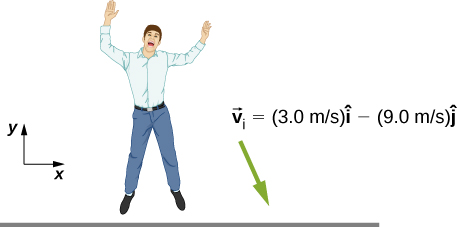1. First find the impulse on the person from the impact on the ground. Calculate both its magnitude and direction.
2. Find the average force on the feet if the person keeps his leg stiff and straight and his center of mass drops by only 1 cm vertically and 1 cm horizontally during the impact.
3. Find the average force on the feet if the person bends his legs throughout the impact so that his center of mass drops by 50 cm vertically and 5 cm horizontally during the impact.
4. Compare the results of part (b) and (c), and draw conclusions about which way is better.

You will need to find the time the impact lasts by making reasonable assumptions about the deceleration. Although the force is not constant during the impact, working with constant average force for this problem is acceptable.

a. $617\,\text{N}\cdot \text{s}$, 108°; b. ${F}_{x}=2.91\times {10}^{4}\,\text{N}$, ${F}_{y}=2.6\times {10}^{5}\,\text{N}$; c. ${F}_{x}=5265\,\text{N}$, ${F}_{y}=5850\,\text{N}$

Two projectiles of mass ${m}_{1}$ and ${m}_{2}$ are ﬁred at the same speed but in opposite directions from two launch sites separated by a distance D. They both reach the same spot in their highest point and strike there. As a result of the impact they stick together and move as a single body afterwards. Find the place they will land.

Two identical objects (such as billiard balls) have a one-dimensional collision in which one is initially motionless. After the collision, the moving object is stationary and the other moves with the same speed as the other originally had. Show that both momentum and kinetic energy are conserved.

Show Solution

Conservation of momentum demands ${m}_{1}{v}_{\text{1,i}}+{m}_{2}{v}_{\text{2,i}}={m}_{1}{v}_{\text{1,f}}+{m}_{2}{v}_{\text{2,f}}$. We are given that ${m}_{1}={m}_{2}$, ${v}_{\text{1,i}}={v}_{\text{2,f}}$, and ${v}_{\text{2,i}}={v}_{\text{1,f}}=0$. Combining these equations with the equation given by conservation of momentum gives ${v}_{\text{1,i}}={v}_{\text{1,i}}$, which is true, so conservation of momentum is satisfied. Conservation of energy demands $\frac{1}{2}{m}_{1}{v}_{\text{1,i}}^{2}+\frac{1}{2}{m}_{2}{v}_{\text{2,i}}^{2}=\frac{1}{2}{m}_{1}{v}_{\text{1,f}}^{2}+\frac{1}{2}{m}_{2}{v}_{\text{2,f}}^{2}$. Again combining this equation with the conditions given above give ${v}_{\text{1,i}}={v}_{\text{1,i}}$, so conservation of energy is satisfied.

A ramp of mass M is at rest on a horizontal surface. A small cart of mass m is placed at the top of the ramp and released.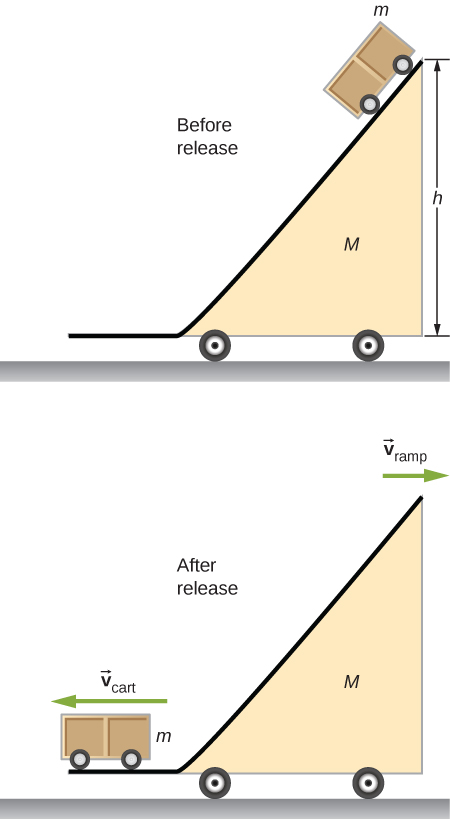What are the velocities of the ramp and the cart relative to the ground at the instant the cart leaves the ramp?

Find the center of mass of the structure given in the figure below. Assume a uniform thickness of 20 cm, and a uniform density of $1{\,\text{g/cm}}^{3}.$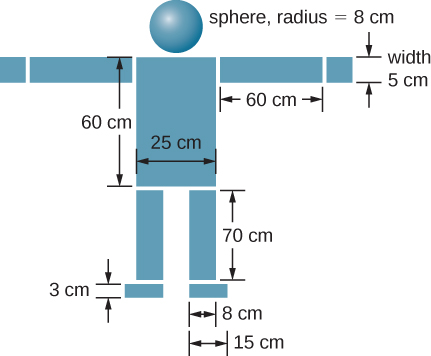Assume origin on centerline and at floor, then $({x}_{\text{CM}},{y}_{\text{CM}})=(0,86\,\text{cm})$

### Glossary

rocket equation
derived by the Soviet physicist Konstantin Tsiolkovsky in 1897, it gives us the change of velocity that the rocket obtains from burning a mass of fuel that decreases the total rocket mass from ${m}_{\text{i}}$ down to m